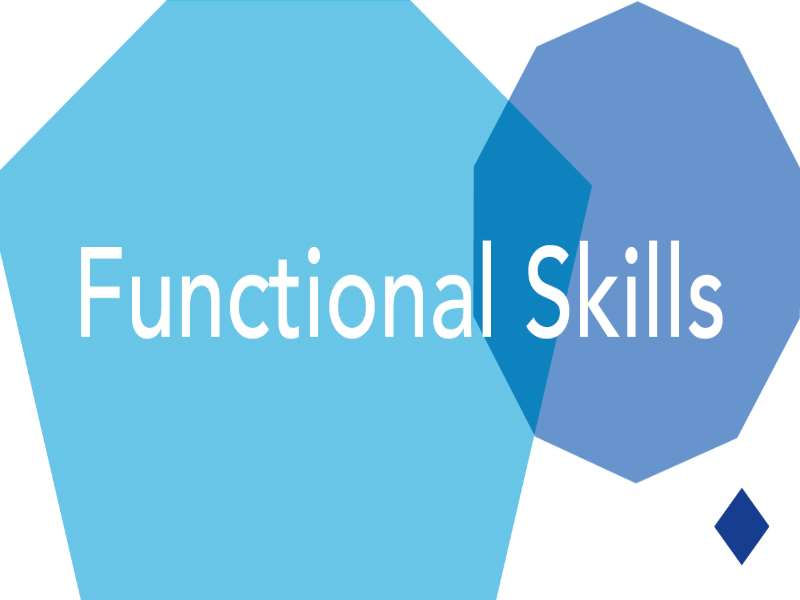Engaging video and e-learning content for social housing and care. e-learning WMB place video presenters into interactive virtual e-learning worlds to produce e-learning that initially looks like a corporate video, but has all the interactivity (and more) of traditional e-learning. Read more...

# Functional Skills Maths (Bundle)(0)
£82.80 per seat

### Overview

Fractions

The course introduces the learner to fractions and the basic methods of manipulating them including adding, dividing, multiplication and division with the use of real life examples applicable the the workplace.

Decimals

The learner will be introduced to decimals and how to calculate them from fractions. Adding, subtracting, multiplication and division will also be taught.

Ratio

The learning covers what ratios are, why we use ration and how to create them from raw data. The course includes worked examples and a quiz.

2D Shapes

This course covers the calculation of area of regular shapes such as squares, rectangles and circles. Examples are given to apply this knowledge to calculate irregular shapes too using work-related examples.

3D Shapes

Learn about calculating the volume of regular 3D shapes such as cylinders and cubes. Examples are given which relate to real life work situations.

Scale

This learning deals with using scale to calculate distances and areas etc. Examples are given of scale drawings and how to use them to make real-life calculations.

Data

This learning covers the organisation of data into data tables as well as the calculation of average and range. Examples of calculating mean, median, mode and range are given.

Probability

Learn how to calculate independent and dependent probabilities using the multiplication and addition of fractions and decision trees .

Charts

Learn more about charts, why they are useful and which charts to use to represent data. The course also includes worked examples of interpreting charts.